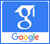# Kinematic pairs## Studies of the possibility of determining amplifications in kinematic pairs

Published on: 11th May, 2020

OCLC Number/Unique Identifier: 8608084505

The article discusses approaches to solving problems of accurately determining strength in kinematic pairs. It is known that the nature of the bonds imposed by kinematic pairs is determined by the geometric shapes of the elements of the pairs. For what, here, the bonds acted during the entire time the mechanism was moving, so that the elements of the kinematic pairs would continuously touch each other. Where it is recognized that one of the simplest methods for taking into account the inertia of a link is the principal moment method. How the contradiction is sought is here because the normal acceleration has a direction opposite because normal acceleration has a direction opposite to the link (directed toward the center), and the image of tangential acceleration is directed parallel to this acceleration. The following simplification can be made if the main vector of inertia is considered together with the weight of the link.